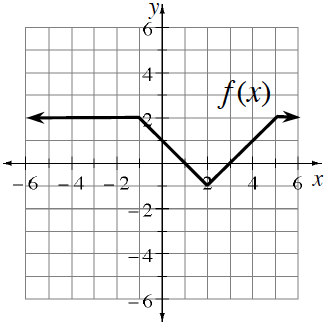### Home > PC3 > Chapter 4 > Lesson 4.1.1 > Problem4-18

4-18.

Using the graph of $y=f(x)$ at right, describe the transformation and sketch a graph of the transformed function.

1. $y=f(x+2)+1$

This transformation is both a horizontal shift and a vertical shift.

1. $y=2f(x)+2$

Order of Operations matters!
Vertically stretch this function first, then apply the vertical shift.

1. $y=-f(x+4)$

This transformation is a vertical reflection and a horizontal shift.